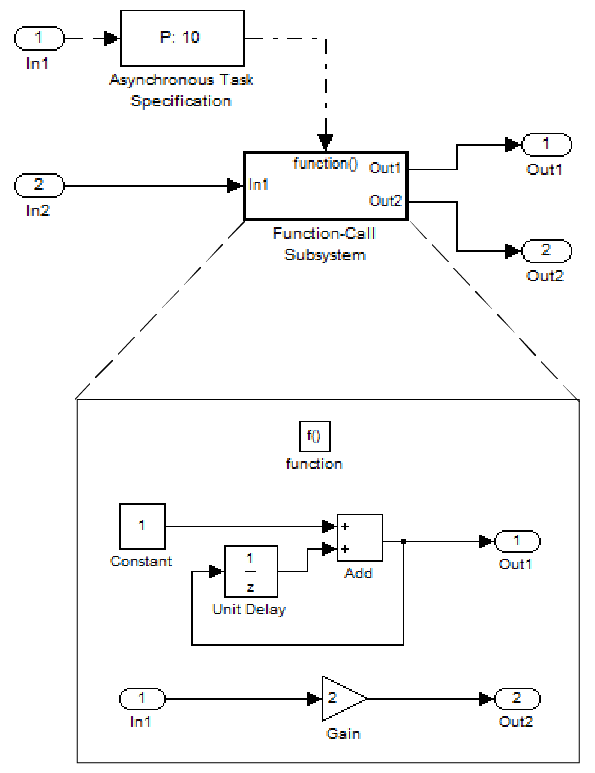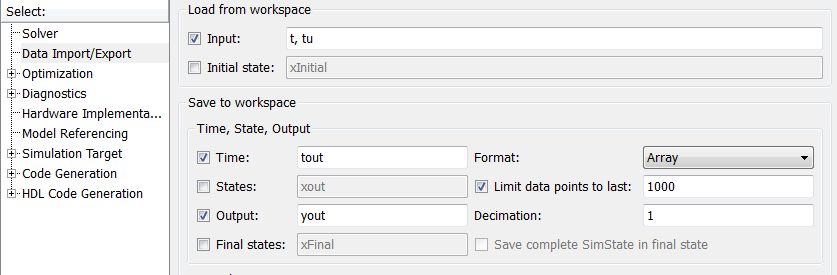## Import Asynchronous Event Data for Simulation

### Capabilities

You can import asynchronous event data into a function-call subsystem via an Inport block. For standalone fixed-step simulations, you can specify:

• The time points at which each asynchronous event occurs

• The number of asynchronous events at each time point

### Input Data Format

You can enter your asynchronous data at the MATLAB® command line or on the Data Import/Export pane of the Configuration Parameters dialog box. In either case, a number of restrictions apply to the data format.

• The expression for parameter model configuration parameter Input must be a comma-separated list of tables.

• The table corresponding to the input port outputting asynchronous events must be a column vector containing time values for the asynchronous events.

• The time vector of the asynchronous events must be of double data type and monotonically increasing.

• Time data must be integer multiples of the model step size.

• To specify multiple function calls at a given time step, you must repeat the time value accordingly. In other words, if you wish to specify three asynchronous events at t = 1 and two events at t = 9, then you must list `1` three times and `9` twice in your time vector. (```t = [1 1 1 9 9]'```)

• The table corresponding to normal data input port can be of another supported format.

### Example

In this model, a function-call subsystem is used to track the total number of asynchronous events and to multiply a set of input values by 2.1. To input data by using the Model Configuration Parameters dialog box,

1. Select Input.

2. For this example, enter the following command in the MATLAB window:

`>> t = [1 1 5 9 9 9]', tu = [[0:10]' [0:10]']`
Alternatively, you can enter the data as t, tu in the Data Import/Export pane:Here, t is a column vector containing the times of asynchronous events for Inport block In1 while tu is a table of input values versus time for Inport block In2.

2. By default, the Time and Output parameters are selected and the output variables are named tout and yout.

3. Simulate the model.

4. Display the output by entering `[tout yout]` at the MATLAB command line and obtain:

```ans = 0 0 -1 1 2 2 2 2 2 3 2 2 4 2 2 5 3 10 6 3 10 7 3 10 8 3 10 9 6 18 10 6 18```

Here the first column contains the simulation times.

The second column represents the output of Out1 — the total number of asynchronous events. Since the function-call subsystem is triggered twice at t = 1, the output is 2. It is not called again until t = 5, and so does not increase to 3 until then. Finally, it is called three times at 9, so it increases to 6.

The third column contains the output of Out2 obtained by multiplying the input value at each asynchronous event time by 2. At any other time, the output is held at its previous value